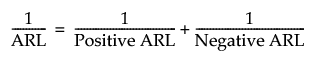Publication date: 11/10/2021

## Average Run Length (ARL) Report

The Average Run Length (ARL) report contains a table and a graph of ARL values. The average run length (ARL) for a specified shift is the average number of runs expected before an out-of-control signal occurs. For example, the ARL at 0 represents the average number of runs expected before seeing a false-alarm signal when the process is in control. When the process is in control, the shift size is 0.

The table and graph in the ARL report enable you to explore how various settings of the parameters affect the performance of the corresponding CUSUM chart. As the h and k parameters in the Control Panel report are updated, the ARL report is updated as well. An ideal CUSUM chart has a high ARL(0) value and a low ARL(Δ) value, where Δ is the size shift that is of interest.

The Average Run Length (ARL) report depends on the settings of the Upper Side and Lower Side options in the Control Panel report. If only one option is selected, the ARL report uses calculations for the corresponding one-sided CUSUM chart. If both options are selected, the ARL report uses calculations for the two-sided CUSUM chart. Note that the two-sided ARL values are related to the positive and negative one-sided ARL values by the following equation:#### ARL Table

The ARL Table shows the average run length for shifts (Δ) between zero and three at 0.25 increments. If the Data Units option is specified, the shift is represented by 2*K/Sigma2. If the Data Units option is not specified, the shift is represented by 2*k/Sigma.

#### ARL Graph

The ARL Graph shows the average run length for shifts (Δ) between 0 and 3. This graph contains the same data points as the ARL Table to the left of the ARL Graph.